Mathematical and Physical Journal
for High Schools
Issued by the MATFUND Foundation
 Already signed up? New to KöMaL?

# KöMaL Problems in Physics, December 2013

Show/hide problems of signs:## Problems with sign 'M'

Deadline expired on January 10, 2014.

M. 337. Measuring the speed of a falling soap bubble, estimate the width of its wall.

(6 pont)

statistics## Problems with sign 'P'

Deadline expired on January 10, 2014.

P. 4583. The skid mark left on the asphalt by a stopping roadster is 290 m. While it was skidding a constant force slowed it down with a deceleration of 3.90 m/s2. What was the speed of the car when it began to skid? What was the magnitude of the force which decelerated it? What was the coefficient of friction between the tires and the asphalt? The total weight of the car with fuel and with its driver is 2150 kg.

(3 pont)

solution (in Hungarian), statistics

P. 4584. Hansel and Gretel have a dream, that they will get married in space. At the end of the ceremony they float next to each other in the space station, but they cannot reach each other, because there is a distance of 10 cm between them. How long do they have to wait until they can reach each other due to gravity? How can they decrease this time? (Estimate the necessary data.)

(4 pont)

solution (in Hungarian), statistics

P. 4585. Two balls of masses M and m are attached to two threads of length, such that the two threads are suspended at the same point. The balls are both deflected to the horizontal position of the threads, released from rest at the same time, and they collide head on. (The collision is perfectly elastic.)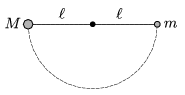a) What should be the M/m ratio of the masses, in order that the ball of mass m reaches the maximum height which is allowed by the thread?

b) In this case how high will the ball of mass M go?

(4 pont)

solution (in Hungarian), statistics

P. 4586. A square shaped, uniform density carpet is rotated by 360o about one of its vertices, such that it slides on the floor with its whole area. The coefficient of kinetic friction is the same at any point of the floor. With performing the same work how many times can this carpet be rotated, sliding on the floor, about its centre?

(5 pont)

solution (in Hungarian), statistics

P. 4587. A pointlike object of mass m is able to move up and down on a vertical fixed rod. There is a vertical hole at the centre of the object, and the rod fits into this hole, such that the object can slide along the rod frictionlessly. Two pieces of thin light threads are attached to the object, and initially the angle between both threads and the vertical is=45o. Each thread goes through a pulley, which are at the same height, and at the other end of each thread an object of mass M=1 kg is tied. The system is released from rest.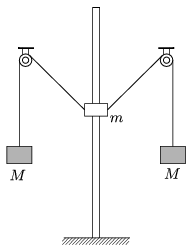a) What is the mass m of the object on the rod, if after releasing the system it stops at the position where the threads attached to it are horizontal?

b) What is the acceleration of the object of mass m when it starts to move back, and what is the acceleration of the other two objects of mass M, when they start to move back?

(4 pont)

solution (in Hungarian), statistics

P. 4588. A uniform density cube of mass m and of side a is placed to a rough horizontal tray, such that along one of its edge it is placed next to a small bumper. The tray is hung by threads above the centre of mass of the cube at a height of(see the figure), and then the system is carefully deflected by some angle, as if it was a pendulum, and then released.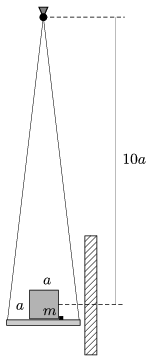When the pendulum arrives back to its lowermost position, the tray collides with a wall of very big mass. What was the angle of deflection if the cube falls through the bumper? The collisions are totally inelastic. (The masses of the tray and the threads are negligible with respect to the mass of the cube. The moment of inertia of the cube around any axis going through the centre of the cube is ma2/6.)

(5 pont)

solution (in Hungarian), statistics

P. 4589. Estimate the distance between the H2O molecules

a) in water at a temperature of 0 oC;

b) in ice at a temperature of 0 oC;

c) in air at a temperature of 0 oC, if the humidity is 100%.

(4 pont)

solution (in Hungarian), statistics

P. 4590. In a thermally insulated cylinder an easily moveable thermally insulated piston separates two samples of air of volumes of 12 l and 8 l at standard condition. There is a heating element in the 8 l volume part, and the temperature of the gas in it is increased to 200 oC.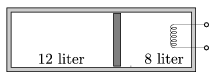a) What are the volumes and the pressures of the two samples of gas at the end of the process?

b) What will the temperature of the gas in the originally 12 l part be at the end of the process?

c) How much heat is absorbed by the gas which is heated?

d) By what amount do the internal energies of the two samples of gas change?

(5 pont)

solution (in Hungarian), statistics

P. 4591. There are 4 positively charged small balls of mass m at the vertices of a regular tetrahedron of edges L. The balls are connected with insulating threads of negligible mass. Three balls have a charge of Q, and the fourth is charged to 2Q.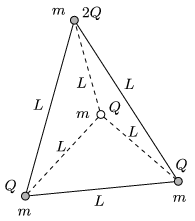a) At the same instant the three threads which connect the charge of 2Q with the others are cut. Determine the initial acceleration of the balls at the instant when the threads are cut.

b) To what speed will the balls accelerate?

(5 pont)

solution (in Hungarian), statistics

P. 4592. There are two conducting wires of negligible resistances in a horizontal plane enclosing an angle of. A long enough rod of mass m is placed onto the wires, perpendicularly to the angle bisector of the angle between the wires at a distance of x0 from the intersection A of the two wires as shown in the figure. The resistance per unit length of the rod is r. The arrangement is put into uniform vertical magnetic field of magnetic induction B.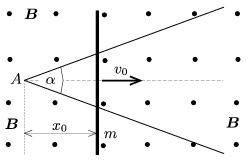The rod is given an initial velocity of v0 in the direction of the angle bisector. The electrical contact between the wires and the rod is good, but the friction is negligible between them. Where will the rod stop?

(5 pont)

solution (in Hungarian), statistics

P. 4593. Two particles whose speeds are very close to the speed of light (ultrarelativistic) collide elastically. The linear momentum vectors of the two particles are shown in the figure. Construct the smallest angle which can be enclosed by the direction of the motion of the two particles flying apart.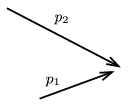(6 pont)

solution (in Hungarian), statistics